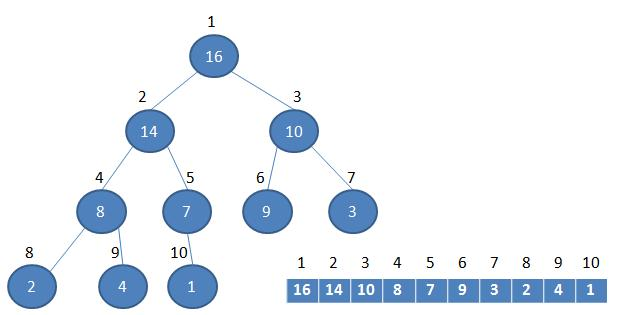# 概述parent(i) = (i-1)/2 向下取整
left(i) = 2i+1
rigth(i) = 2i+2# Java代码实现

/**
* 堆排序实现数组排序, 从小到大顺序
* @param arr
*/
public static void heapSort(int[] arr) {
/**
* 1. 将堆调整为最大堆
* 2. 将堆顶元素与末尾元素交换
* 3. 重新调整堆, 并将堆顶元素与末尾元素交换
* 4. 重复执行第三步, 直到只剩一个元素
*/
// 构建最大堆, 从第一个非叶子结点向前, 依次进行构建, 最终生成最大堆
for (int i = arr.length / 2 - 1; i >= 0; i--) {
// 从第一个非叶子结点从下至上，从右至左调整结构
}
// 进行堆排序
for (int j = arr.length - 1; j > 0; j--) {
// 将堆顶元素与末尾元素进行交换
int tmp = arr;
arr = arr[j];
arr[j] = tmp;
// 重新对堆进行调整,将最大值提到堆顶
}

}

/**
* 调整最大堆, 假设子结构 最大堆已经构建
*
* @param arr
* @param i
*          需要调整的结点
* @param length
*          当前数组的长度
*/
private static void adjustHeap(int[] arr, int i, int length) {
/**
* 1. 从当前结点的左子结点开始查找
* 2. 若右子结点大就使用右子节点
* 3. 若子结点大于当前元素, 令子结点往上冒
* 4. 循环找到最后的大于当前元素的叶子结点
* 5. 将当前元素放到为他空出来的位置
*/
// 先取出当前元素i
int tmp = arr[i];
// 从i结点的左子结点开始，也就是2i+1处开始
for (int k = i * 2 + 1; k < length; k = k * 2 + 1) {
// 如果左子结点小于右子结点，k指向右子结点
if (k + 1 < length && arr[k] < arr[k + 1]) {
k++;
}
// 如果子节点大于当前元素, 令其往上冒
if (arr[k] > tmp) {
arr[i] = arr[k];
i = k;
} else {
break;
}
}
// 将temp值放到最终的位置
arr[i] = tmp;
}0 评论

0